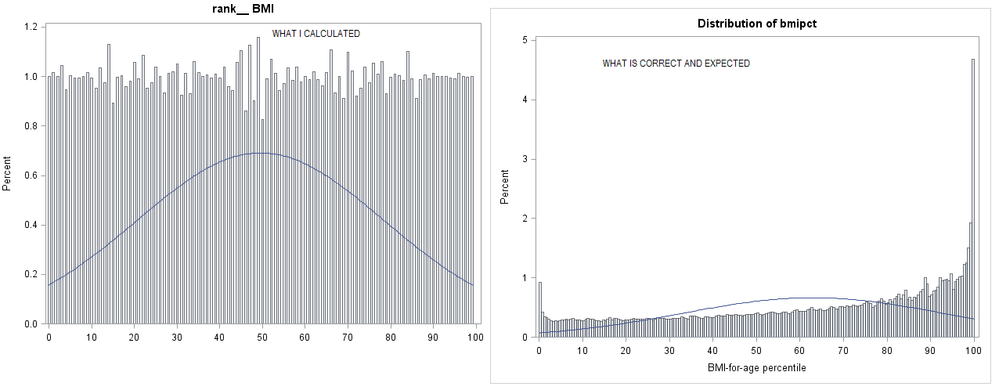## Creating continuous percentile value for all observations in dataI'd like to calculate percentile of BMI of all observation (person)  in data. Below is my attempt. I'm puzzled with resulting histogram on percentile (rank_BMI) . People with higher BMI percentile are at higher risk of being obese et.c. However, how is it possible that all percentiles are uniformly distributed 😞  Correct and expected "percentile of BMI for age" is also shown in the image below @ballardw

`````` proc rank data=Mydata groups=100 out=ranked;
var BMI;
ranks rank_BMI;
run;

proc univariate data=ranked noprint;
histogram rank_BMI/
normal(noprint)
nocurvelegend;
label rank_BMI="pctl of BMI";
run;``````

1 ACCEPTED SOLUTION

Accepted Solutions

## Re: Creating continuous percentile value for all observations in data

The issue with the shown plot is that you are sort of misunderstanding what the Proc Ranks is doing. Please see this result from your code on some data with two different distributions.

```data example;
do i= 1 to 10000;
val = rand('normal',0,1);
val2 = rand('exponential');
output;
end;
run;

proc rank data=example groups=100 out=ranked;
var val val2;
ranks rank_val rank_val2;
run;

proc univariate data=ranked noprint ;
histogram rank_val/
normal(noprint)
nocurvelegend;
histogram val/
normal(noprint)
nocurvelegend;
label val='raw data normal';
label rank_val="pctl of normal VAL";
histogram rank_val2/
normal(noprint)
nocurvelegend;
histogram val2/
normal(noprint)
nocurvelegend;
label val2='raw data exponential';
label rank_val2="pctl of exponential VAL2";
run;
```

Depending a number of setting your univariate graph of rank_val may look a bit odd depending on the number of bins univariate displays. The question I would actually ask is more why isn't your percentile actually flatter.

What does the histogram of your BMI variable look like?

3 REPLIES 3

## Re: Creating continuous percentile value for all observations in data

I failed to customize this solution to my data. Macro didn't work for me.

https://blogs.sas.com/content/sgf/2017/07/21/selecting-the-top-n-and-bottom-n-of-observations-from-a...

## Re: Creating continuous percentile value for all observations in data

The issue with the shown plot is that you are sort of misunderstanding what the Proc Ranks is doing. Please see this result from your code on some data with two different distributions.

```data example;
do i= 1 to 10000;
val = rand('normal',0,1);
val2 = rand('exponential');
output;
end;
run;

proc rank data=example groups=100 out=ranked;
var val val2;
ranks rank_val rank_val2;
run;

proc univariate data=ranked noprint ;
histogram rank_val/
normal(noprint)
nocurvelegend;
histogram val/
normal(noprint)
nocurvelegend;
label val='raw data normal';
label rank_val="pctl of normal VAL";
histogram rank_val2/
normal(noprint)
nocurvelegend;
histogram val2/
normal(noprint)
nocurvelegend;
label val2='raw data exponential';
label rank_val2="pctl of exponential VAL2";
run;
```

Depending a number of setting your univariate graph of rank_val may look a bit odd depending on the number of bins univariate displays. The question I would actually ask is more why isn't your percentile actually flatter.

What does the histogram of your BMI variable look like?

## Re: Creating continuous percentile value for all observations in data

Percentiles by definition break the data up into uniform groups.

I don't think you want a histogram, I think you want the CDF from PROC UNIVARIATE on the raw data based on your comments. It does not match your graph though.

Discussion stats
• 3 replies
• 1037 views
• 3 likes
• 3 in conversation